# Introduction to Equivalence Testing with TOSTER

#### 2023-04-05

For an open access tutorial paper explaining how to set equivalence bounds, and how to perform and report equivalence tests, see Lakens, D., Scheel, A. M., & Isager, P. M. (2018). Equivalence Testing for Psychological Research: A Tutorial. Advances in Methods and Practices in Psychological Science, 1(2), 259-269. https://doi.org/10.1177/2515245918770963

Warning: many of the TOSTER functions within this particular vignette are outdated. Where this is the case, we have included the updated code as well. Please see the other vignettes for details on the new functions. The “Introduction to t_TOST” vignette, in particular, will be helpful. This vignette, and the functions used, will continue to be in the package for continuity purposes.

# Equivalence Testing with TOSTER

Scientists should be able to provide support for the absence of a meaningful effect. Currently researchers often incorrectly conclude an effect is absent based a non-significant result. It is statistically impossible to support the hypothesis that a true effect size is exactly zero. What is possible in a Frequentist hypothesis testing framework is to statistically reject effects large enough to be deemed worthwhile. When researchers want to argue for the absence of an effect that is large enough to be worthwhile to examine, they can test for equivalence.

A widely recommended approach within a Frequentist framework is to test for equivalence using the TOST procedure (Schuirmann, 1987), because of its simplicity and widespread use in other scientific disciplines. The goal in the TOST approach is to specify a lower and upper bound, such that results falling within this range are deemed equivalent to the absence of an effect that is worthwhile to examine (e.g., $$\Delta$$L = -0.3 to $$\Delta$$U = 0.3, where $$\Delta$$ is a difference that can be defined by either standardized differences such as Cohen’s d, or raw differences such as 0.3 scale point on a 5-point scale). In the TOST procedure the null hypothesis is the presence of a true effect of $$\Delta$$L or $$\Delta$$U, and the alternative hypothesis is an effect that falls within the equivalence bounds, or the absence of an effect that is worthwhile to examine. The observed data is compared against $$\Delta$$L and $$\Delta$$U in two one-sided tests. If the p-value for both tests indicates the observed data is surprising, assuming $$\Delta$$L or $$\Delta$$U are true, we can follow a Neyman-Pearson approach to statistical inferences and reject effect sizes larger than the equivalence bounds. When making such a statement, we will not be wrong more often, in the long run, than our Type 1 error rate (e.g., 5%).

In this document I will present a number of practical examples for equivalence tests for independent t-tests, dependent t-tests, one-sample t-tests, correlations, and meta-analyses.

# Equivalence test for Meta-analysis

Hyde, Lindberg, Linn, Ellis, and Williams (2008) report that effect sizes for gender differences in mathematics tests across the 7 million students in the US represent trivial differences, where a trivial difference is specified as an effect size smaller then d = 0.1. The present a table with Cohen’s d and se is reproduced below:

library("TOSTER")
TOSTmeta(ES = 0.06, se = 0.003, low_eqbound_d=-0.1, high_eqbound_d=0.1, alpha=0.05)
## TOST results:
## Z-value lower bound: 53.33   p-value lower bound: 0.000
## Z-value upper bound: -13.33  p-value upper bound: 0.00000000000000000000000000000000000000007
##
## Equivalence bounds (Cohen's d):
## low eqbound: -0.1
## high eqbound: 0.1
##
## TOST confidence interval:
## lower bound 90% CI: 0.055
## upper bound 90% CI:  0.065
##
## NHST confidence interval:
## lower bound 95% CI: 0.054
## upper bound 95% CI:  0.066
##
## Equivalence Test Result:
## The equivalence test was significant, Z = -13.333, p = 0.0000000000000000000000000000000000000000741, given equivalence bounds of -0.100 and 0.100 and an alpha of 0.05.
## 
##
## Null Hypothesis Test Result:
## The null hypothesis test was significant, Z = 20.000, p = 0.0000000000000000000000000000000000000000000000000000000000000000000000000000000000000000551, given an alpha of 0.05.
## 
## NHST: reject null significance hypothesis that the effect is equal to 0
## TOST: reject null equivalence hypothesis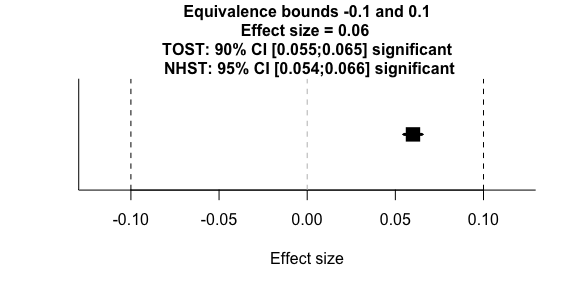We see that indeed, all effect size estimates are measured with such high precision, we can conclude that they fall within the equivalence bound of d = -0.1 and d = 0.1. However, note that all of the effects are also statistically significant - so, the the effects are statistically different from zero, and practically equivalent.

Hyde, J. S., Lindberg, S. M., Linn, M. C., Ellis, A. B., & Williams, C. C. (2008). Gender similarities characterize math performance. Science, 321(5888), 494-495.

# Independent Groups Equivalence Test

## Independent Groups Student’s Equivalence Test

Eskine (2013) showed that participants who had been exposed to organic food were substantially harsher in their moral judgments relative to those exposed to control (d = 0.81, 95% CI: [0.19, 1.45]). A replication by Moery & Calin-Jageman (2016, Study 2) did not observe a significant effect (Control: n = 95, M = 5.25, SD = 0.91, Organic Food: n = 89, M = 5.22, SD = 0.83). Following Simonsohn’s (2015) recommendation the equivalence bound was set to the effect size the original study had 33% power to detect (with n = 21 in each condition, this means the equivalence bound is d = 0.48, which equals a difference of 0.429 on a 7-point scale given the sample sizes and a pooled standard deviation of 0.894). Using a TOST equivalence test with alpha = 0.05, assuming equal variances, and equivalence bounds of d = -0.48 and d = 0.48 is significant, t(182) = -3.03, p = 0.001. We can reject effects more extreme than d = 0.48 (or a raw difference of 0.429 scalepoints).

# OLD CODE
#TOSTtwo(m1=5.25,m2=5.22,sd1=0.95,sd2=0.83,n1=95,n2=89,low_eqbound_d=-0.48, high_eqbound=0.48, alpha = 0.05, var.equal=TRUE)
TOSTtwo.raw(m1=5.25,m2=5.22,sd1=0.95,sd2=0.83,n1=95,n2=89,low_eqbound=-0.429, high_eqbound=0.429, alpha = 0.05, var.equal=TRUE)
## Note: this function is defunct. Please use tsum_TOST instead
## TOST results:
## t-value lower bound: 3.48    p-value lower bound: 0.0003
## t-value upper bound: -3.03   p-value upper bound: 0.001
## degrees of freedom : 182
##
## Equivalence bounds (raw scores):
## low eqbound: -0.429
## high eqbound: 0.429
##
## TOST confidence interval:
## lower bound 90% CI: -0.188
## upper bound 90% CI:  0.248
##
## NHST confidence interval:
## lower bound 95% CI: -0.23
## upper bound 95% CI:  0.29
##
## Equivalence Test Result:
## The equivalence test was significant, t(182) = -3.025, p = 0.00142, given equivalence bounds of -0.429 and 0.429 (on a raw scale) and an alpha of 0.05.
## 
##
## Null Hypothesis Test Result:
## The null hypothesis test was non-significant, t(182) = 0.227, p = 0.820, given an alpha of 0.05.
## 
## NHST: don't reject null significance hypothesis that the effect is equal to 0
## TOST: reject null equivalence hypothesis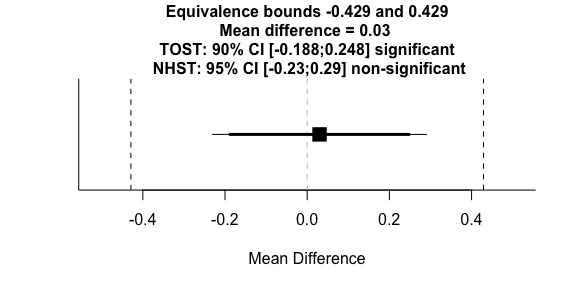# NEW CODE
tsum_TOST(m1=5.25,m2=5.22,sd1=0.95,sd2=0.83,n1=95,n2=89,eqb=0.429, alpha = 0.05, var.equal=TRUE)
##
## Standard Two-Sample t-Test
##
## The equivalence test was significant, t(182) = -3.025, p = 1.42e-03
## The null hypothesis test was non-significant, t(182) = 0.227, p = 8.2e-01
## NHST: don't reject null significance hypothesis that the effect is equal to zero
## TOST: reject null equivalence hypothesis
##
## TOST Results
##                  t  df p.value
## t-test      0.2275 182    0.82
## TOST Lower  3.4804 182 < 0.001
## TOST Upper -3.0254 182   0.001
##
## Effect Sizes
##            Estimate     SE             C.I. Conf. Level
## Raw         0.03000 0.1319  [-0.188, 0.248]         0.9
## Hedges's g  0.03342 0.1469 [-0.2083, 0.275]         0.9
## Note: SMD confidence intervals are an approximation. See vignette("SMD_calcs").

Thanks to Brent Donnelan for pointing out the SD in the control condition in the replication was 0.91, not 0.95 as in an earlier version of this vignette, and the raw equivalence bound is 0.429, not 0.384 as in an earlier version. I mistook the n (95) and the SD, and originally calculated raw bounds for 0.43, not 0.48 by mistake.

Moery, E., & Calin-Jageman, R. J. (2016). Direct and Conceptual Replications of Eskine (2013): Organic Food Exposure Has Little to No Effect on Moral Judgments and Prosocial Behavior. Social Psychological and Personality Science, 7(4), 312-319. https://doi.org/10.1177/1948550616639649

## Independent Groups Welch’s Equivalence Test

Deary, Thorpe, Wilson, Starr, and Whally (2003) report the IQ scores of 79,376 children in Scotland (39,343 girls and 40,033 boys). The IQ score for girls (M = 100.64, SD = 14.1) girls and boys (100.48, SD = 14.9) was non-significant. With such a huge sample, we can examine whether the data is equivalent using very small equivalence bounds, such as -0.05 and 0.05. Because sample sizes are unequal, and variances are unequal, Welch’s t-test is used, and we can conclude IQ scores are indeed equivalent, based on equivalence bounds of -0.05 and 0.05.

# OLD CODE
TOSTtwo(m1=100.64,m2=100.48,sd1=14.1,sd2=14.9,n1=39343,n2=40033,low_eqbound_d=-0.05, high_eqbound_d=0.05, alpha = 0.05, var.equal=FALSE)
## Note: this function is defunct. Please use tsum_TOST instead
## TOST results:
## t-value lower bound: 8.60    p-value lower bound: 0.000000000000000004
## t-value upper bound: -5.49   p-value upper bound: 0.00000002
## degrees of freedom : 79260.94
##
## Equivalence bounds (Cohen's d):
## low eqbound: -0.05
## high eqbound: 0.05
##
## Equivalence bounds (raw scores):
## low eqbound: -0.7253
## high eqbound: 0.7253
##
## TOST confidence interval:
## lower bound 90% CI: -0.009
## upper bound 90% CI:  0.329
##
## NHST confidence interval:
## lower bound 95% CI: -0.042
## upper bound 95% CI:  0.362
##
## Equivalence Test Result:
## The equivalence test was significant, t(79260.94) = -5.491, p = 0.0000000201, given equivalence bounds of -0.725 and 0.725 (on a raw scale) and an alpha of 0.05.
## 
##
## Null Hypothesis Test Result:
## The null hypothesis test was non-significant, t(79260.94) = 1.554, p = 0.120, given an alpha of 0.05.
## 
## NHST: don't reject null significance hypothesis that the effect is equal to 0
## TOST: reject null equivalence hypothesis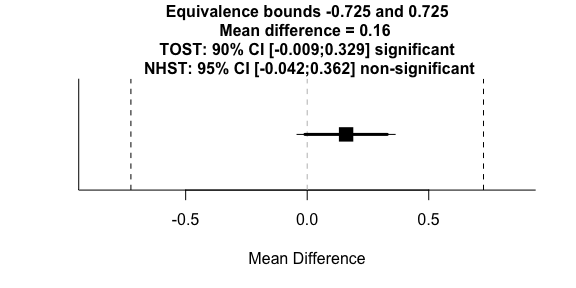# NEW CODE
tsum_TOST(m1=100.64,m2=100.48,sd1=14.1,sd2=14.9,n1=39343,n2=40033,eqb=0.05, alpha = 0.05, var.equal=FALSE,
eqbound_type = "SMD")
## Warning: setting bound type to SMD produces biased results!
##
## Welch Modified Two-Sample t-Test
##
## The equivalence test was significant, t(79260.94) = -5.491, p = 2.01e-08
## The null hypothesis test was non-significant, t(79260.94) = 1.554, p = 1.2e-01
## NHST: don't reject null significance hypothesis that the effect is equal to zero
## TOST: reject null equivalence hypothesis
##
## TOST Results
##                 t    df p.value
## t-test      1.554 79261    0.12
## TOST Lower  8.599 79261 < 0.001
## TOST Upper -5.491 79261 < 0.001
##
## Effect Sizes
##                Estimate       SE              C.I. Conf. Level
## Raw             0.16000 0.102951 [-0.0093, 0.3293]         0.9
## Hedges's g(av)  0.01103 0.007098  [-6e-04, 0.0227]         0.9
## Note: SMD confidence intervals are an approximation. See vignette("SMD_calcs").

Deary, I. J., Thorpe, G., Wilson, V., Starr, J. M., & Whalley, L. J. (2003). Population sex differences in IQ at age 11: The Scottish mental survey 1932. Intelligence, 31(6), 533-542.

# One-Sample Equivalence Test

Lakens, Semin, & Foroni (2012) examined whether the color of Chinese ideographs (white vs. black) would bias whether participants judged that the ideograph correctly translated positive, neutral, or negative words. In Study 4 the prediction was that participants would judge negative words to be correctly translated by black ideographs above guessing average, but no effects were predicted for translations in the other 5 between subject conditions in the 2 (ideograph color: white vs. black) x 3 (word meaning: positive, neutral, negative) design. The table below is a summary of the data in all 6 conditions:

Color Valence M % SD t df p d
White Positive 6.05 50.42 1.50 0.15 20 .89 .03
White Negative 6.68 55.67 1.70 1.75 18 .10 .40
White Neutral 5.95 49.58 1.40 0.87 19 .87 .04
Black Positive 6.45 53.75 1.91 1.06 19 .30 .24
Black Negative 6.95 57.92 1.15 3.71 19 .00 .80
Black Neutral 5.71 47.58 1.79 0.73 20 .47 .16

With 19 to 21 participants in each between subject condition, the study had 80% power to detect equivalence with equivalence bounds of -0.68 to d = 0.68.

# OLD CODE
powerTOSTone(alpha=0.05, statistical_power=0.8, low_eqbound_d=-0.68, high_eqbound_d=0.68)
## Note: this function is defunct. Please use power_t_TOST instead
## The required sample size to achieve 80 % power with equivalence bounds of -0.68 and 0.68 is 19
## 
##  18.52043
# NEW CODE -- note there is a minor discrepancy
# because the new function uses a different solution for power
power_t_TOST(type = "one.sample",eqb = 0.68,
power = 0.8,alpha=.05)
##
##      One-sample TOST power calculation
##
##           power = 0.8
##            beta = 0.2
##           alpha = 0.05
##               n = 19.9539
##           delta = 0
##              sd = 1
##          bounds = -0.68, 0.68

When we perform 5 equivalence tests using equivalence bounds of -0.68 and 0.68, using a Holm-Bonferroni sequential procedure to control error rates, we can conclude statistical equivalence for the data in row 1, 3, and 6, but the conclusion is undetermined for tests 2 and 4, where the data is neither significantly different from guessing average, nor statistically equivalent. For example, performing the test for the data in row 6:

# OLD CODE
TOSTone(m=5.71,mu=6,sd=1.79,n=20,low_eqbound_d=-0.68, high_eqbound_d=0.68, alpha=0.05)
## Note: this function is defunct. Please use tsum_TOST instead
## TOST results:
## t-value lower bound: 2.32    p-value lower bound: 0.016
## t-value upper bound: -3.77   p-value upper bound: 0.0007
## degrees of freedom : 19
##
## Equivalence bounds (Cohen's d):
## low eqbound: -0.68
## high eqbound: 0.68
##
## Equivalence bounds (raw scores):
## low eqbound: -1.2172
## high eqbound: 1.2172
##
## TOST confidence interval:
## lower bound 90% CI: -0.982
## upper bound 90% CI:  0.402
##
## NHST confidence interval:
## lower bound 95% CI: -1.128
## upper bound 95% CI:  0.548
##
## Equivalence Test Result:
## The equivalence test was significant, t(19) = 2.317, p = 0.0159, given equivalence bounds of -1.217 and 1.217 (on a raw scale) and an alpha of 0.05.
## 
##
## Null Hypothesis Test Result:
## The null hypothesis test was non-significant, t(19) = -0.725, p = 0.478, given an alpha of 0.05.
## 
## NHST: don't reject null significance hypothesis that the effect is equal to 0
## TOST: reject null equivalence hypothesis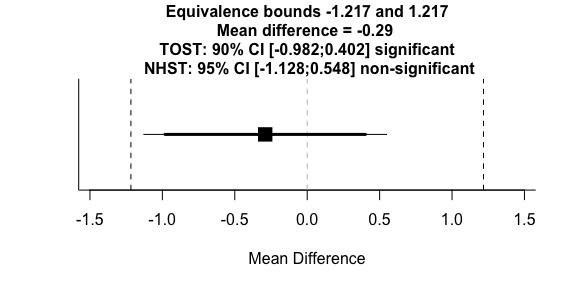# NEW CODE
tsum_TOST(m1=5.71-6,sd1=1.79,n1=20,eqb=0.68, eqbound_type = "SMD")
## Warning: setting bound type to SMD produces biased results!
##
## One-sample t-Test
##
## The equivalence test was significant, t(19) = 2.317, p = 1.59e-02
## The null hypothesis test was non-significant, t(19) = -0.725, p = 4.78e-01
## NHST: don't reject null significance hypothesis that the effect is equal to zero
## TOST: reject null equivalence hypothesis
##
## TOST Results
##                  t df p.value
## t-test     -0.7245 19   0.478
## TOST Lower  2.3165 19   0.016
## TOST Upper -3.7656 19 < 0.001
##
## Effect Sizes
##            Estimate     SE              C.I. Conf. Level
## Raw         -0.2900 0.4003 [-0.9821, 0.4021]         0.9
## Hedges's g  -0.1555 0.2250   [-0.509, 0.202]         0.9
## Note: SMD confidence intervals are an approximation. See vignette("SMD_calcs").

It is clear I should have been more tentative in my conclusions in this study. Not only can I not conclude equivalence in some of the conditions, the equivalence bound I had 80% power to detect is very large, meaning the possibility that there are theoretically interesting but smaller effects remains.

Lakens, D., Semin, G. R., & Foroni, F. (2012). But for the bad, there would not be good: Grounding valence in brightness through shared relational structures. Journal of Experimental Psychology: General, 141(3), 584-594. DOI: 10.1037/a0026468

# Equivalence test for correlations

Olson, Fazio, and Hermann (2007) reported correlations between implicit and explicit measures of self-esteem, such as the IAT, Rosenberg’s self-esteem scale, a feeling thermometer, and trait ratings. In Study 1 71 participants completed the self-esteem measures. Because no equivalence bounds are mentioned, we can see which equivalence bounds the researchers would have 80% power to detect.

# No new code for correlations (yet)
powerTOSTr(alpha=0.05, statistical_power=0.8, low_eqbound_r=-0.24, high_eqbound_r=0.24)
## The required sample size to achieve 80 % power with equivalence bounds of -0.24 and 0.24 is 146 observations
## 
##  145.9348

With 71 pairs of observations between measures, the researchers have 80% power for equivalence bounds of r = -0.24 and r = 0.24.

The correlations observed by Olson et al (2007), Study 1, are presented in the table below (significant correlations are flagged by an asteriks).

Measure IAT Rosenberg Feeling thermometer Trait ratings
IAT - -.12 -.09 -.06
Rosenberg - .62* .09
Feeling thermometer - .29*
Trait ratings -

We can test each correlation, for example the correlation between the IAT and the Rosenberg self-esteem scale of -0.12, given 71 participants, and using equivalence bounds of r_U = 0.24 and r_L = -0.24.

# OLD CODE
TOSTr(n=71, r=-0.12, low_eqbound_r=-0.24, high_eqbound_r=0.24, alpha=0.05)
## TOST results:
## p-value lower bound: 0.153
## p-value upper bound: 0.001
##
## Equivalence bounds (r):
## low eqbound: -0.24
## high eqbound: 0.24
##
## TOST confidence interval:
## lower bound 90% CI: -0.31
## upper bound 90% CI:  0.079
##
## NHST confidence interval:
## lower bound 95% CI: -0.344
## upper bound 95% CI:  0.117
##
## Equivalence Test Result:
## The equivalence test was non-significant, p = 0.153, given equivalence bounds of -0.240 and 0.240 and an alpha of 0.05.
## 
##
## Null Hypothesis Test Result:
## The null hypothesis test was non-significant, p = 0.319, given an alpha of 0.05.
## 
## NHST: don't reject null significance hypothesis that the correlation is equal to 0
## TOST: don't reject null equivalence hypothesis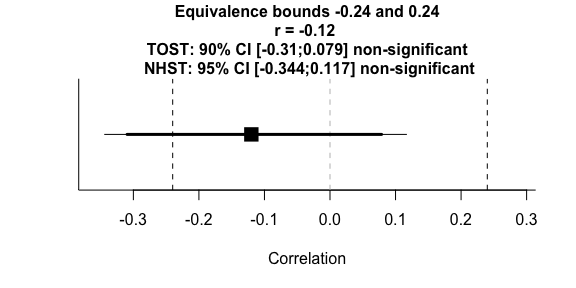# NEW CODE
corsum_test(n=71, r=-0.12, null=0.24, alpha=0.05,
alternative = "equivalence")
##
##  Pearson's product-moment correlation
##
## data:  x and y
## z = 1.0241, N = 71, p-value = 0.1529
## alternative hypothesis: equivalence
## null values:
## correlation correlation
##        0.24       -0.24
## 90 percent confidence interval:
##  -0.30955107  0.07872354
## sample estimates:
##   cor
## -0.12

We see that none of the correlations can be declared to be statistically equivalent. Instead, the tests yield undetermined outcomes: the correlations are not significantly different from 0, nor statistically equivalent.

Olson, M. A., Fazio, R. H., & Hermann, A. D. (2007). Reporting tendencies underlie discrepancies between implicit and explicit measures of self-esteem. Psychological Science, 18(4), 287-291.